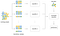# Random Forest — A Democratic Voting System

Machine Learning algorithms for regression and classification problems can be handled by a powerful tool called decision tree methods. This is the second article about decision tree methods where I will be discussing about Random Forest.https://www.alliance-scotland.org.uk/blog/news/weekly-radio-show-shines-spotlight-disability-sports/

On my previous article, I discussed about the core of Cart, Classification and Regression Tree, as well as the ways for finding the best cut-point to split where the cost function is minimum, Gini, Entropy and RSS calculations and I finalized my article with the pros and cons of Cart.

In order to reach Random Forest, we’ll first explore two important concepts: Bagging and Random Subspace.

If the training data is being split randomly and two models are being constructed with these two parts, the models would be quite different from each other. This high variance should be eliminated and there comes the bagging method!

Bagging, which is a short term for Bootstrap Aggregation, was proposed by Leo Breiman.

Bagging predictors is a method for generating multiple versions of a predictor and using these to get an aggregated predictor. The aggregation averages over the versions when predicting a numerical outcome and does a plurality vote when predicting a class. 

It is a general-purpose procedure for reducing the variance of a statistical learning method. In order to reduce the variance, therefore increase the prediction accuracy of the model and bring a democratic voting system, several samples are being collected from the dataset and separate models are being built with these different samples.

So, how do we split the dataset and pick samples?

With Bootstrap method, the dataset is being split randomly into n samples and theoretically each sample represents a different part of the dataset.

In the below graphic, the dataset contain various information (visualized with yellow, green and blue dots). Each sample being picked from the dataset contain different ratios of dots (a different pattern). When several models are being built with these sample datasets, each resulting model will be successful for accurately predicting a different part of the dataset. When averaging the set of models, we will be able to have an average model that has learned all the patterns within the dataset. So long high variance and welcome the wisdom of the crowd!https://pinard.medium.com/classification-and-regression-tree-cart-b2d61412b60b

The logic behind this method is very similar to bagging. Bagging randomly samples the observations whereas Random Subspace randomly samples the features for each model. So all the features of the dataset is the space and samples of the features are subspaces. By selecting features, the number of dimensions within the dataset is reduced and different sets of features (all features define the model in a different way) are being gathered.

This method has been proposed by Tin Kam Ho.

# Random Forest

Random Forest, a versatile method proposed by Breiman, is a supervised learning algorithm that can be used for both regression and classification problems. It consists of a large number of decision trees, so there is an ensemble of trees having different backgrounds and a democratic voting system.

With Bagging and Random Subspace approaches, features and observations are being sampled randomly and several models are being built. It is important to note that the resulting tree models are independent, carrying the pattern of the randomly picked part of the dataset. This random sampling method enables the model to average all the patterns and therefore be able to represent the whole dataset. This randomizing and ensemble approaches prevent the model from over-fitting, which generally occurs in Cart.

Just like Cart, Random Forest is robust to outliers and missing values. The only exception can be the outliers in the outcome in regression problems.

1. Data Science and Machine Learning Bootcamp Lectures https://www.veribilimiokulu.com/bootcamp-programlari/veri-bilimci-yetistirme-programi/
2. Gareth James, Daniela Witten, Trevor Hastie, Robert Tibshirani. An Introduction to Statistical Learning : with Applications in R. New York :Springer, 2013.
3. Breiman, L. Bagging predictors. Mach Learn 24, 123–140 (1996)
4. https://mathchi.medium.com/weak-learners-strong-learners-for-machine-learning-e73e32f86ebd
5. https://pinard.medium.com/classification-and-regression-tree-cart-b2d61412b60b